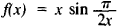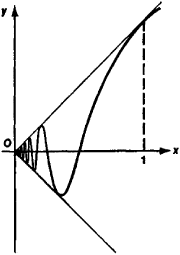# length

(redirected from crown-rump length)
Also found in: Dictionary, Thesaurus, Medical, Wikipedia.
Related to crown-rump length: biparietal diameter

## length

1. Prosody Phonetics the metrical quantity or temporal duration of a vowel or syllable
2. the distance from one end of a rectangular swimming bath to the other
3. Prosody the quality of a vowel, whether stressed or unstressed, that distinguishes it from another vowel of similar articulatory characteristics. Thus in English beat is of greater length than in English bit
4. Cricket the distance from the batsman at which the ball pitches
5. Bridge a holding of four or more cards in a suit

## Length

A one-dimensional extension in space. Length is one of the three fundamental physical quantities, the others being mass and time. It can be measured by comparison with an arbitrary standard; the specific one in most common usage is the international meter. In 1983, at the meeting of the Conférence Général des Poids et Mésures, the meter was redefined in terms of time and the speed of light: “The meter is the length of the path traveled by light in vacuum during a time interval of 1/299 792 458 of a second.” This definition defines the speed of light to be exactly 299 792 458 m/s, and defines the meter in terms of the most accurately known quantity, the second. See Light, Mass, Time

## Length

a numerical characteristic of the extent of curves. The concept of length is defined differently for different cases. (1) The length of a line segment is the distance between its end points, measured by some segment taken as a unit of length. (2) The length of a polygonal line is the sum of the lengths of its components. (3) The length of a simple arc is the limit of the lengths of polygonal lines inscribed in the arc, when the number of components increases indefinitely and the maximum length of the components tends to zero. (4) The length of a continuous curve consisting of a finite number of simple arcs is equal to the sum of the lengths of these arcs. For example, the circumference of a circle can be obtained as the limit of the perimeters of inscribed regular polygons when the number of their sides is doubled indefinitely; it is equal to 2πR where R is the radius of the circle. Any continuous curve has finite or infinite length. If its length is finite, then the curve is said to be rectifiable. The graph (see Figure 1) of the functionfor 0 <x ≤ 1 and f(x) = 0 when x = 0 is an example of a nonrectifiable curve; here the lengths of the inscribed polygonal lines increase beyond all bound as the length of the components tend to zero. If the equation of a plane curve has the form y =f(x) (a ^ x ^ b)m rectangular coordinates and the function f(x) has a continuous derivative f’(x), then the length of the curve is given by the integralThe length of a curve given in parametric form and the length of a space curve can be expressed in a similar manner.Figure 1

To calculate the length of a curve the mathematicians of antiquity essentially used lengths of polygonal lines and passage to the limit. For them, however, such passage to the limit was only a method for calculating the length of a curve and not for defining the concept of the length of a curve, since they apparently perceived the latter as one of the elementary mathematical concepts. The necessity of defining the length of a curve became clear only in the first half of the 19th century. A full elucidation of the problem was achieved by C. Jordan. In differential geometry the length of a curve is also defined on a surface or in an arbitrary Riemannian space.

### REFERENCES

Lebesgue, H. Ob izmerenii velichin, 2nd ed. Moscow, 1960. (Translated from French.)
Fikhtengol’ts, G. M. Kurs differentsial’nogo i integral’nogo ischisleniya, 7th ed. vol. 2. Moscow, 1969.

## length

[leŋkth]
(mechanics)
Extension in space.
References in periodicals archive ?
Wildschut et al., "First-trimester crown-rump length and embryonic volume of aneuploid fetuses measured in virtual reality," Ultrasound in Obstetrics and Gynecology, vol.
CRL = crown-rump length, NO = nasooccipital length, tail = tail length, and waist [empty set] = waist diameter.
This diagnosis was based on a symptom-generated ultrasound that showed an empty gestational sac; a gestational sac with a yolk sac but no embryo when the mean gestational sac diameter was less than 20 mm or less than 30 mm; or an embryo with an absent heart beat and a crown-rump length of less than 6 mm or less than 8 mm.
In the case of adiponectin, regression analysis demonstrated that the serum concentration was significantly affected by maternal age, weight, cigarette smoking status, and racial origin but not method of conception (P = 0.637), parity (P = 0.219), or fetal crown-rump length (P = 0.459), as expressed in the following regression equation: square-root adiponectin expected = 130.19 + 0.74 x maternal age in years + (-18.24 if the racial origin was African, -31.89 if South Asian, 0 if Caucasian, East Asian, or mixed) -0.53 x maternal weight in kg -10.38 if cigarette smoker ([R.sup.2] = 0.223, P < 0.0001).
Provide participants with the tools and knowledge needed to evaluate and interpret nuchal translucency and crown-rump length measurements 6.
First-trimester screening characteristics Screen negative Variable N=356 Range Age of patient (yrs) 30.1 18233 Gestational age (wks.d) 13.2 11.1-14.0 Crown-rump length (mm) 69.4 65-84 Fetal heart rate (beats/min) 164 146-180 Nuchal translucency (mm) 1.8 1.0-2.5 Correct recall of LNMP (%) 46 Screen positive Variable N=59 Range p-value Age of patient (yrs) 31 23-43 0.466 Gestational age (wks.d) 12.5 11.2-13.6 <0.01 Crown-rump length (mm) 64.7 47.0-83.2 0.01 Fetal heart rate (beats/min) 170 147-185 0.34 Nuchal translucency (mm) 3.65 1.7-9.6 <0.01 Correct recall of LNMP (%) 23 <0.01 LNMP = last normal menstrual period.
Of the five females taken on 15-16 May 2004, one was lactating and the other four were pregnant with single embryos that averaged 26.3 (23-32) in crown-rump length. Eight females were captured during our work between 13 and 17 July 2002.
Statistical tests used were analysis of variance (ANOVA) for litter size, analysis of covariance (ANCOVA) using litter size as a covariate for newborn weight and crown-rump length, implantation sites, and resorptions, and repeated-measures ANCOVA for weight changes during pregnancy, where weight of the mother at GD6, 9, 12, 15, and 18 was the repeated measure and weight at GDO was the covariate.
The physician can perform on-screen measurements of fetal anatomy, such as crown-rump length, head circumference, and length of the femur, and can then use these measurements to calculate the gestational age.
These included thigh length, leg length, upper arm length, forearm length, bi-iliocristal diameter, bitrochanteric diameter, transverse chest diameter, chest depth, biacromial diameter, head length, head breadth, chest circumference, upper arm circumference, calf circumference, biocular breadth, bizygomatic breadth, bigonial breadth, upper face height, trunk length, and crown-rump length. All measurements were reported in millimeters (O'Rourke 1980).
Endovaginal US: demonstration of cardiac activity in embryos of less than 5.0 mm in crown-rump length. Radiology 1990;176(1):71-4.
The number of male and female fetuses was counted; for each fetus, the placenta and body weight were recorded, and fetal crown-rump length was measured.

Site: Follow: Share:
Open / Close